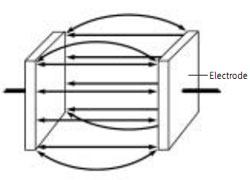Conductivity Theory

Background

Electrical conductivity is an inherent property of most materials, and ranges from extremely conductive materials like metals to highly resistive materials such as polymers or glass. Aqueous solutions, such as sea water and plating baths exhibit conductivity between these two extremes. In aqueous solutions, the electrical current is carried by charged ions. The conductivity is determined by the number of charge carriers, how fast they move, and how much charge each one carries. Hence, for most aqueous solutions, the higher the concentration of dissolved salts, which will lead to more ions, the higher the conductivity. This effect continues until the solution gets to a maximum value, after which, the conductivity may actually decrease with increasing concentration. This can result in two different concentrations of a salt having the same conductivity. This needs to be considered when using conductivity to determine concentrations of solutions. Some species ionize more completely in water (strong electrolytes such as NaCl & HCl)  than others do, and their solutions are more conductive as a result. Each acid, base, or salt has its own characteristic curve for concentration vs. conductivity. Raising the temperature gives more energy to the ions making them move faster, and hence increasing the conductivity.. Since ions are of different sizes, and carry different amounts of water with them as they move, the temperature effect is different for each ion. Typically, the conductivity varies about 1–3% per degree C, and this temperature coefficient may itself vary with concentration and temperature.

Definitions

The conductivity of a material is an inherent property. Pure water at a particular temperature will always have the same conductivity. The conductance of a sample of pure water depends on how the measurement is made—how big a sample, how far apart the measuring electrodes are, etc. It is defined as the reciprocal of the resistance in ohms, measured between the opposing faces of a 1 cm cube of liquid at a specific temperature. See Conductivity Cell Figure. The basic unit of conductance is the Siemens (S).Because a measurement gives the conductance, techniques have been worked out to convert the measured value to the conductivity, so that results can be compared from different experiments. This is done by measuring a cell constant (K) for each setup, using a solution of known conductivity.
Cell Conductance x Cell Constant (K) = Conductivity
(Equation 1)

The cell constant is related to the physical characteristics of the measuring cell. K is defined for two flat, parallel measuring electrodes as the electrode separation distance (d) divided by the electrode area (A). Thus, for a 1 cm cube of liquid:-

K = d/A = 1 cm–1
(Equation 2)In practice, the measured cell value is entered into the meter, and the conversion from conductance to conductivity is done automatically. The K value used varies with the linear measuring range of the cell selected. Typically, a cell with K = 0.1 cm–1 is chosen for pure water measurements, while for environmental water and industrial solutions a cell with K of 0.4 to 1 cm–1 is used. Cells with up to K = 10 cm–1 are best for very high conductivity samples. For some solutions, such as pure water, the conductivity numbers are so low that some users prefer to use resistivity and resistance instead. The resistivity is the reciprocal of the conductivity (R = 1/C), and the resistance is the reciprocal of the conductance. Resistance units are in ohms, and 1 ohm = 1/Siemens. From Eq. 1 and 2, it can be seen that conductivity units are in Siemens/cm, and therefore resistivity units are in ohm–cm. The resistivity of ultrapure water is 18 megaohm-cm.

How is conductivity measured?

In the simplest arrangement (a 2-electrode cell), a voltage is applied to two flat plates immersed in the solution, and the resulting current is measured.  From Ohm’s Law, the conductance = current/voltage. Actually there are many practical difficulties. Solution conductivity is due to ion mobility. Using  DC voltage would soon deplete the ions near the plates, causing polarization, and a higher than actual resistance. This can be mostly overcome by using AC voltage, but then the instrument designer must correct for various capacitance and other effects. Modern sophisticated 2-electrode conductivity instruments use complex AC waveforms to minimize these effects, and by using the cell constant, measured temperature, temperature coefficient and reference temperature, will report sample conductivity.

Durability

Cell materials should be chosen to fit the needs of the application. For field and demanding laboratory applications, many users choose a cellconstructed with an epoxy body and carbon measuring electrodes, as this has been shown to be extremely durable and chemically resistant. For especially demanding applications, some manufacturers supply probe guards, which can be attached to the probe for additional protection. For pure water applications such as USP injectable water testing, stainless steel is the material of choice. It is very durable, can be manufactured to precise tolerances, and for low conductivity, low contact resistances are not required.For chemically reactive samples, glass and platinum are frequently the optimum choice, as they have the best overall chemical resistance of all commonly used cell materials

Table 1 Typical Conductivities of Common Solutions
 Sample at 25 °C Conductivity, µS/cm Ultrapure Water 0.055 Distilled Water 1.0 Rain Water 50 Drinking Water 500 Industrial Wastewater 5,000 Seawater 50,000 1M NaCl 85,000 1M HCl 332,000

Table 2 - TC (Temperature Coefficient Values)
 Sample Percent/°C (at 25 °C) Ultrapure Water 4.55 Salt solution (NaCl) 2.12 5% NaOH 1.72 Dilute Ammonia Solution 1.88 10% HCl 1.32 5% Sulfuric Acid 0.96 98% Sulfuric Acid 2.84 Sugar Syrup 5.64

By Type
By Application
Theory
By Parameter